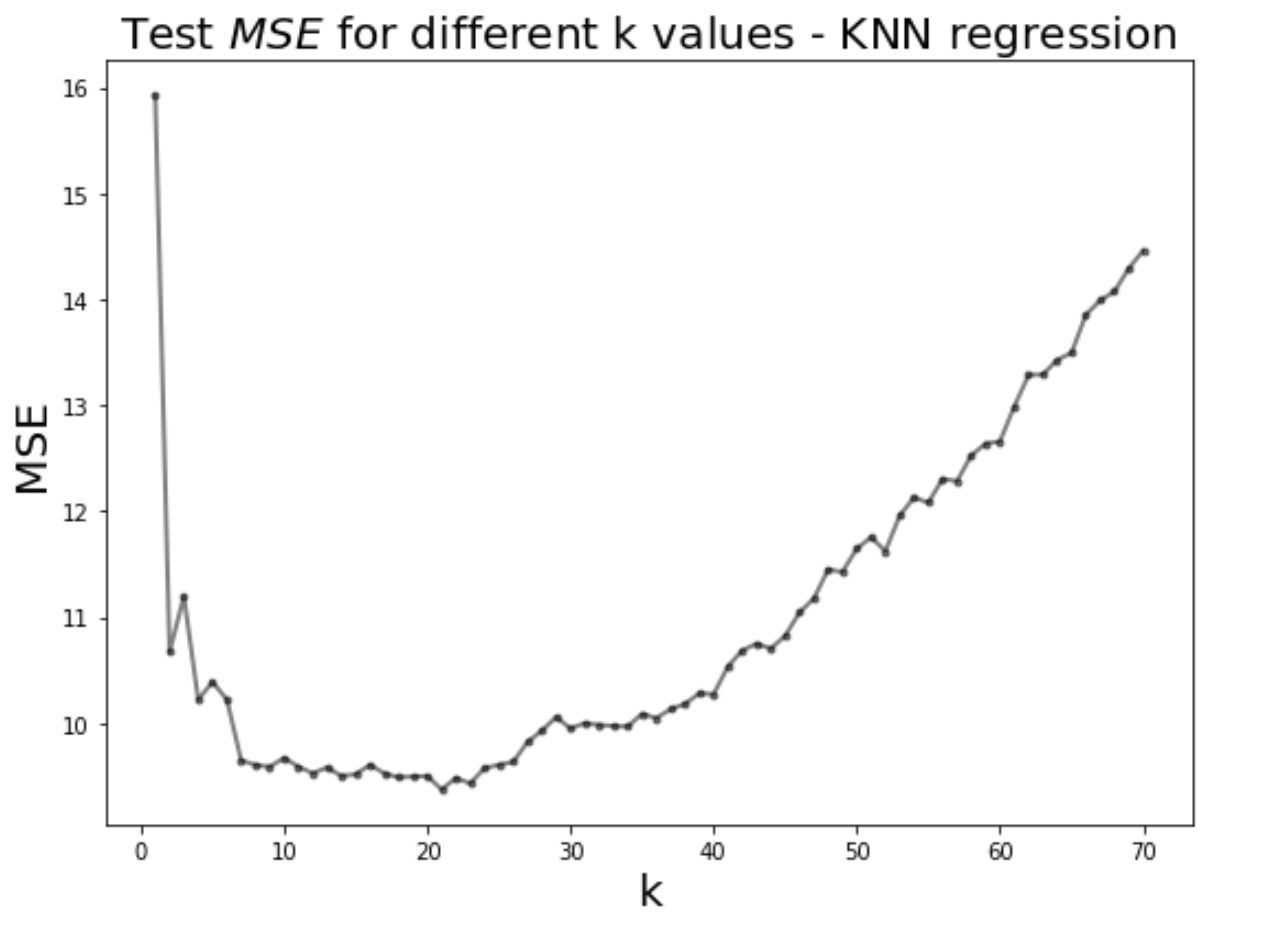# Title¶

Exercise: B.1 - Finding best k in kNN regression

# Description¶

The goal here is to find the value of k of the best performing model based on the test MSE.# Instructions:¶

• Select the sales column as the response variable and TV budget column as the predictor variable
• Make a train-test split using sklearn.model_selection.train_test_split
• Create a list of integer k values using numpy.linspace
• For each value of k
• Fit a knn regression on train set
• Calculate MSE on test set and store it
• Plot the test MSE values for each k
• Find the k value associated with the lowest test MSE

# Hints:¶

train_test_split(X,y) : Split arrays or matrices into random train and test subsets.

np.linspace() : Returns evenly spaced numbers over a specified interval.

KNeighborsRegressor(n_neighbors=k_value) : Regression-based on k-nearest neighbors.

mean_squared_error() : Computes the mean squared error regression loss.

dict.keys() : returns a view object that displays a list of all the keys in the dictionary.

dict.values() : returns a list of all the values available in a given dictionary.

dict.items() : returns a list of dict's (key, value) tuple pairs

Note: This exercise is auto-graded and you can try multiple attempts.

In :
import pandas as pd
import numpy as np
from sklearn.model_selection import train_test_split
from sklearn.utils import shuffle
from sklearn.neighbors import KNeighborsRegressor
from sklearn.metrics import mean_squared_error
import matplotlib.pyplot as plt
from sklearn.metrics import r2_score

%matplotlib inline


In [ ]:
# Data set used in this exercise


In [ ]:
# again, take a quick look at the data


In :
# Select the 'TV' column as predictor variable and 'Sales' column as response variable

x = df[[___]]
y = df[___]


### Train-Test split¶

In :
### edTest(test_shape) ###
# Split the dataset in training and testing with 60% training set and 40% testing set

x_train, x_test, y_train, y_test = train_test_split(___,___,train_size=___,random_state=66)

In :
### edTest(test_nums) ###
# Choosing k range from 1 to 70
k_value_min = 1
k_value_max = 70

# creating list of integer k values betwwen k_value_min and k_value_max using linspace
k_list = np.linspace(k_value_min,k_value_max,num=70,dtype=int)


### Model fit¶

In :
# hint mean_squared_error

In [ ]:
fig, ax = plt.subplots(figsize=(10,6))

# creating a dictionary for storing k value against MSE fit {k: MSE@k}

knn_dict = {}
j=0
# Looping over k values
for k_value in k_list:

# creating KNN Regression model
model = KNeighborsRegressor(n_neighbors=int(___))

# fitting model
model.fit(x_train,y_train)

# predictions
y_pred = model.predict(___)

# Calculating MSE
MSE = ____

#Storing the MSE values of each k value in a dictionary
knn_dict[k_value] = ___

## Plotting
colors = ['grey','r','b']
if k_value in [1,10,70]:
xvals = np.linspace(x.min(),x.max(),100)
ypreds = model.predict(xvals)
ax.plot(xvals, ypreds,'-',label = f'k = {int(k_value)}',linewidth=j+2,color = colors[j])
j+=1

ax.legend(loc='lower right',fontsize=20)
ax.plot(x_train, y_train,'x',label='test',color='k')
ax.set_xlabel('TV budget in $1000',fontsize=20) ax.set_ylabel('Sales in$1000',fontsize=20)
plt.tight_layout()


### Graph plot¶

In [ ]:
# Plot k against MSE

plt.figure(figsize=(8,6))
plt.plot(___, ___,'k.-',alpha=0.5,linewidth=2)

plt.xlabel('k',fontsize=20)
plt.ylabel('MSE',fontsize = 20)
plt.title('Test $MSE$ values for different k values - KNN regression',fontsize=20)
plt.tight_layout()


### Find the best knn model¶

In [ ]:
### edTest(test_mse) ###

# Looking for k with minimum MSE

min_mse = min(___)
best_model = ___       # HINT YOU MAY USE LIST COMPREHENSION
print ("The best k value is ",best_model,"with a MSE of ", min_mse)


## How good is your model?¶

### From the options below, how would you classify your model?¶

1. Good
2. Satisfactory
In [ ]:
# Your answer here

In [ ]:
# Run this cell to calculate the R2_score of your best model
model = KNeighborsRegressor(n_neighbors=best_model)
model.fit(x_train,y_train)
y_pred_test = model.predict(x_test)

print(f"The R2 score for your model is {r2_score(y_test, y_pred_test)}")


### After observing the $R^2$ value, how would you now classify your model?¶

1. Good
2. Satisfactory
# your answer here